# Mass Weight and Density Quiz 1

#### Quiz Description

Here in this quiz, we shall go through some exciting concepts in physics which are: Mass, Weight, and Density. These three are very much related as mass is used in calculations involving weight and density. This quiz is tailored towards the ability to measure the mass of a substance, measure the volume of a substance, calculate the weight of a body, as well as the volume of that body or any given fluid.

Mass is the amount of matter contained in a body. It could also be described to be in direct relationship with the inertia of a body (The reluctance of a body to change its velocity). On the other hand, weight can be defined to be the force which pulls a body towards the earth.

Density on the other hand could be defined as the mass per unit volume of a body or fluid, commonly measured in kilograms per cubic meter (kg/m^3).

This quiz among others, is one of the other A-level physics quizzes made available for you. Do you want to fully understand the ideas behind mass, weight, and density? All you need to do is take the quiz and see how much you know and need to know. Good luck as you practice for your exams.

A brick is placed on a newton meter and then on a beam balance.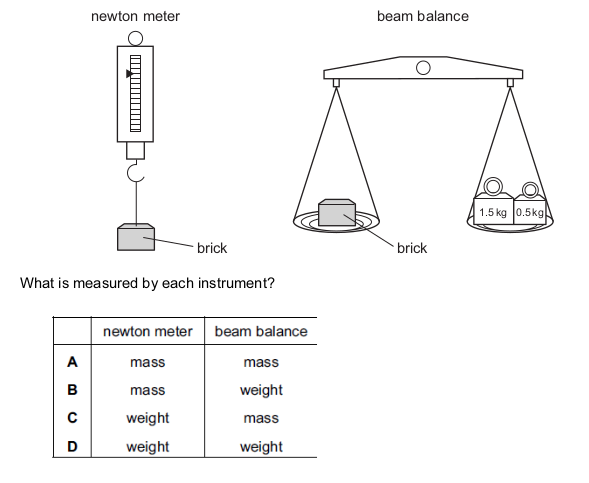Correct
• A
• B
• C
• D

Which property of a body resists change from a state of rest or of motion?

Correct
• density
• mass
• volume
• weight

The mass of a stone is found on Earth using a pan balance. The weight of the stone is found using a newton meter.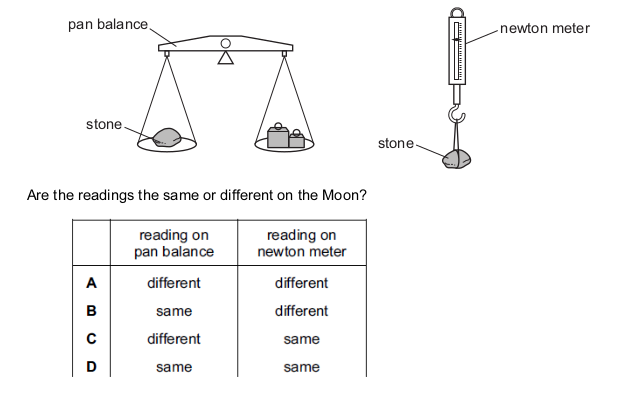Correct
• A
• B
• C
• D

The table shows the weights and masses of four objects on different planets.

On which planet is the gravitational field strength the largest?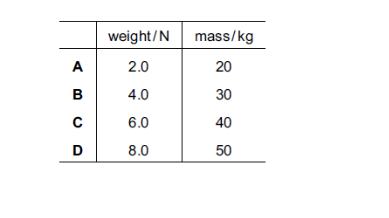Correct
• A
• B
• C
• D

The mass and volume of four different objects are plotted as shown.

Which object has the smallest density?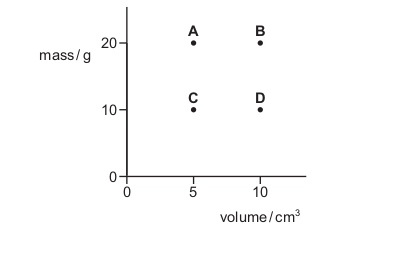Correct
• A
• B
• C
• D

Which statement about mass is correct?

Correct
• Mass is density divided by volume.
• Mass is the amount of space occupied by a body.
• Mass is weight multiplied by the gravitational field strength.
• Mass resists a change in motion.

A student produces some revision notes on gravity, mass and weight.

Which statement is not correct?

Correct
• A gravitational field is a region in which a mass experiences a gravitational force.
• Gravitational field strength has the unit of N / kg.
• Mass is a measure of the amount of matter.
• Mass is equal to weight multiplied by gravitational field strength.

Mass is equal to weight multiplied by gravitational field strength.

The height of P is twice the height of Q. The diameter of P is half the diameter of Q.

Which statement is correct?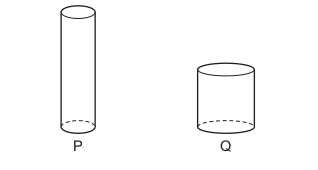Correct
• The density of cylinder P is four times that of cylinder Q.
• The density of cylinder P is twice that of cylinder Q.
• The density of cylinder P is equal to that of cylinder Q.
• The density of cylinder P is half that of cylinder Q.

Four objects are situated in places with different gravitational field strengths.

Which object has the greatest weight?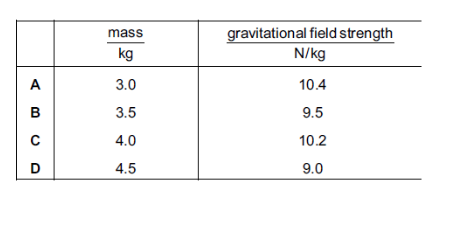Correct
• A
• B
• C
• D

Four rocks on different planets have masses and weights as shown.

Which planet has the greatest gravitational field strength?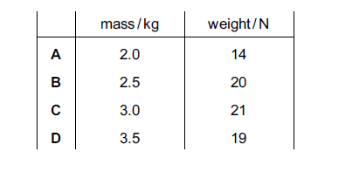Correct
• A
• B
• C
• D

A stone has a mass of 390 g and a density of 2.7 g / cm 3. Cooking oil has a density of 0.90 g / cm 3.

Which mass of oil has the same volume as the stone?

Correct
• 130 g
• 160 g
• 900 g
• 1200 g

A scientist places a rock on a spring balance. She then places the same rock on a beam balance.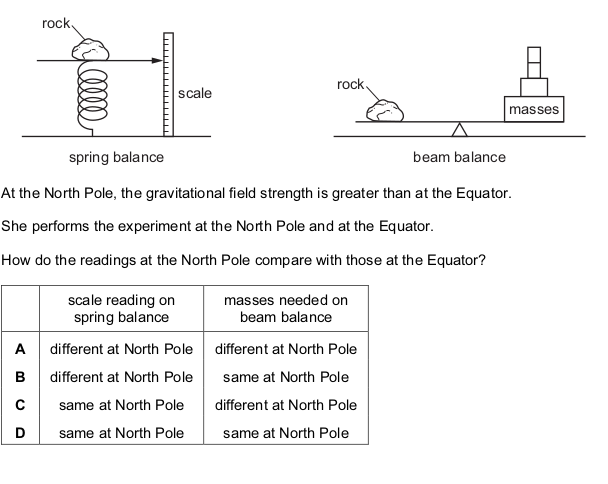Correct
• A
• B
• C
• D

A boy stands on some bathroom scales. The reading on the scales is 50 kg.

What is the mass and what is the weight of the boy?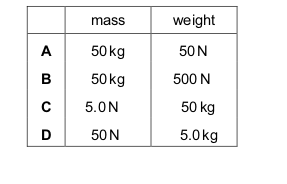Correct
• A
• B
• C
• D

Two identical beakers contain the same mass of liquid. There is a different liquid in each beaker.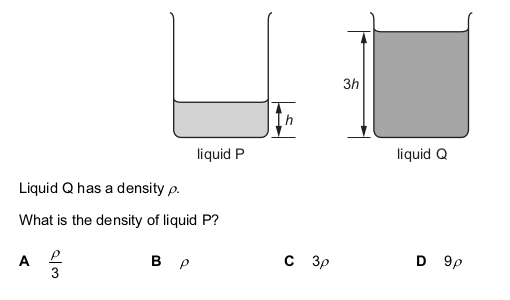Correct
• A
• B
• C
• D

Paper is sold in packets labelled 80 g / m 2 . This means that a sheet of paper of area 10 000 cm 2 has a mass of 80 g. The thickness of each sheet is 0.11 mm.

What is the density of the paper?

Correct
• 0.073 g / cm 3
• 0.088 g / cm 3
• 0.73 g / cm 3
• 0.88 g / cm 3

A measuring cylinder contains 20 cm 3 of water. A stone is placed in the water and the water level rises to 38 cm 3 .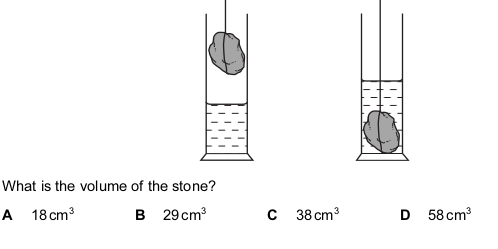Correct
• A
• B
• C
• D

A spacecraft travels from the Earth to the Moon. At a certain point in the journey, it has zero weight.

Why is the weight zero at this point?

Correct
• The gravitational fields of the Earth and the Moon cancel each other.
• The spacecraft leaves the Earth’s gravitational field.
• The spacecraft stops moving.
• There is no air resistance on the spacecraft.

The weight of a cylinder on the Moon is less than its weight on the Earth. How do the gravitational field strength and the mass of the cylinder on the Moon compare with their values on the Earth?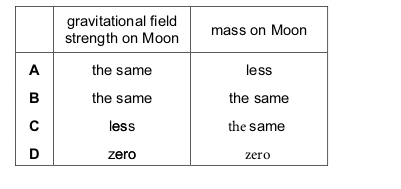Correct
• A
• B
• C
• D

A block of metal is taken from the Earth to the Moon. Which property of the block changes?

Correct
• density
• mass
• volume
• weight

The mass of a paper-clip is 0.50 g and the density of its material is 8.0 g / cm 3 . The total volume of a number of clips is 20 cm 3.

How many paper-clips are there?

Correct
• 80
• 160
• 240
• 320

The mass and the volume of a bar made from metal X are measured.

The masses and volumes of four other bars are measured.

Which bar is made from a metal with a density that is double that of X?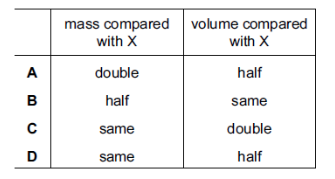Correct
• A
• B
• C
• D